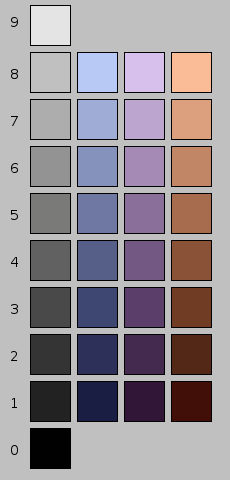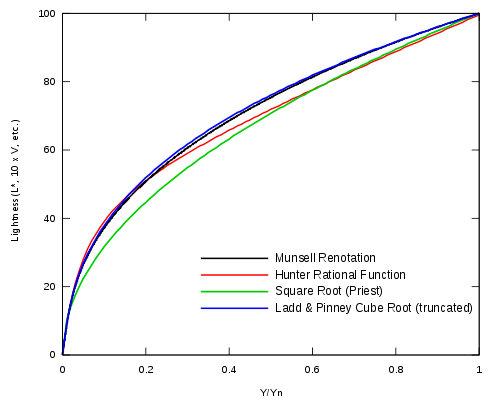# Lightness (color)

﻿
Lightness (color)Three hues in the Munsell color model. Each color differs in value from top to bottom in equal perception steps. The right column undergoes a dramatic change in perceived color.

Lightness (sometimes called value or tone) is a property of a color, or a dimension of a color space, that is defined in a way to reflect the subjective brightness perception of a color for humans along a lightness–darkness axis. A color's lightness also corresponds to its amplitude.

Various color models have an explicit term for this property. The Munsell color model uses the term value, while the HSL color model and Lab color space use the term lightness. The HSV model uses the term value a little differently: a color with a low value is nearly black, but one with a high value is the pure, fully saturated color.

In subtractive color (i.e. paints) value changes can be achieved by adding black or white to the color. However, this also reduces saturation. Chiaroscuro and Tenebrism both take advantage of dramatic contrasts of value to heighten drama in art. Artists may also employ shading, subtle manipulation of value.

## Relationship between lightness, value, and luminance

The Munsell value has long been used as a perceptually uniform lightness scale. A question of interest is the relationship between the Munsell value scale and the relative luminance. Aware of the Weber–Fechner law, Munsell remarked "Should we use a logarithmic curve or curve of squares?" Neither option turned out to be quite correct; scientists eventually converged on a roughly cube-root curve, consistent with the Stevens power law for brightness perception, reflecting the fact that lightness is proportional to the number of nerve impulses per nerve fiber per unit time. The remainder of this section is a chronology of lightness approximations, leading to CIELAB.

Note: Munsell's V runs from 0 to 10, while Y typically runs from 0 to 100 (often interpreted as a percent). Typically, the relative luminance is normalized so that the "reference white" (say, magnesium oxide) has a tristimulus value of Y=100. Since the reflectance of magnesium oxide (MgO) relative to the perfect reflecting diffuser is 97.5%, V=10 corresponds to Y=100/97.5%≈102.6 if MgO is used as the reference.Observe that the lightness is 50% for a luminance of around 18% relative to the reference white.
1920
Priest et al. provide a basic estimate of the Munsell value (with Y running from 0 to 1 in this case):$V=10 \sqrt{Y}$
1933
Munsell, Sloan, and Godlove launch a study on the Munsell neutral value scale, considering several proposals relating the relative luminance to the Munsell value, and suggest:
V2 = 1.4742Y − 0.004743Y2
1943
Newhall, Nickerson, and Judd prepare a report for the Optical Society of America. They suggest a quintic parabola (relating the reflectance in terms of the value):
Y = 1.2219V − 0.23111V2 + 0.23951V3 − 0.021009V4 + 0.0008404V5
1943
Using Table II of the O.S.A. report, Moon and Spencer express the value in terms of the luminance:
V = 5(Y / 19.77)0.426 = 1.4Y0.426
1944
Saunderson and Milner introduce a subtractive constant in the previous expression, for a better fit to the Munsell value. Later, Jameson and Hurvich claim that this corrects for simultaneous contrast effects.
V = 2.357Y0.343 − 1.52
1955
Ladd and Pinney of Eastman Kodak are interested in the Munsell value as a perceptually uniform lightness scale for use in television. After considering one logarithmic and five power-law functions (per Stevens' power law), they relate value to reflectance by raising the reflectance to the power of 0.352:
V = 2.217Y0.352 − 1.324
Realizing this is quite close to the cube root, they simplify it to:
V = 2.468Y1 / 3 − 1.636
1958
Glasser et al. define the lightness as ten times the Munsell value (so that the lightness ranges from 0 to 100):
L * = 25.29Y1 / 3 − 18.38
1964
Wyszecki simplifies this to:
W * = 25Y1 / 3 − 17
This formula approximates the Munsell value function for 1% < Y < 98% (it is not applicable for Y<1%) and is used for the CIE 1964 color space.
1976
CIELAB uses the following formula:
L * = 116(Y / Yn)1 / 3 − 16
where Yn is the Y tristimulus value of a "specified white object" and is subject to the restriction Y / Yn > 0.01. Pauli removes this restriction by computing a linear extrapolation which maps Y/Yn=0 to L*=0 and is tangent to the formula above at the point at which the linear extension takes effect. First, the transition point is determined to be$Y/Y_n=(6/29)^3 \approx 0.008856$, then the slope of$(29/3)^3 \approx 903.3$ is computed. This gives the two-part function:$f(u)= \left\{\begin{array}{ll}\frac{841}{108}u + \frac{4}{29}, & u \le (6/29)^3 \\ \\ u^{1/3}, & u > (6/29)^3\end{array}\right.$
The lightness is then L * = 116f(Y / Yn) − 16.

At first glance, you might approximate the lightness function by a cube root, an approximation that is found in much of the technical literature. However, the linear segment near black is significant. The best-fit pure power function has an exponent of about 0.42, far from 1/3.

An 18% grey card, having a reflectance of 0.18, has lightness very close to 50. It is called "mid grey" because its lightness is midway between black and white.

Wikimedia Foundation. 2010.

### Look at other dictionaries:

• Color constancy — Color constancy: The colors of a hot air balloon are recognized as being the same in sun and shade …   Wikipedia

• Lightness (disambiguation) — Lightness may refer to: *Lightness, a property of a color. *An object or material with relatively small mass, weight or density. *Lightness, a philosophical concept most closely associated with continental philosophy and existentialism, which is… …   Wikipedia

• Lightness — Light ness, n. [From {Light} bright.] 1. Illumination, or degree of illumination; as, the lightness of a room. Chaucer. [1913 Webster] 2. Absence of depth or of duskiness in color; as, the lightness of a tint; lightness of complexion. [1913… …   The Collaborative International Dictionary of English

• Color analysis (art) — Color analysis, also called skin tone color matching or color seasons, is the process of finding colors of clothing and makeup to match a person s complexion, eye color, and hair color. It is often used as an aid to wardrobe planning and style… …   Wikipedia

• color — [kul′ər] n. [ME & OFr colour < L color < OL colos, orig., a covering < IE base * kel , to conceal, hide > HULL1, HALL] 1. the sensation resulting from stimulation of the retina of the eye by light waves of certain lengths 2. the… …   English World dictionary

• Color (manga) — Color Cover of Color as published by Shinshokan. Genre Yaoi Manga …   Wikipedia

• Color theory — For the American electronica/synthpop musician, see Color Theory. In the visual arts, color theory is a body of practical guidance to color mixing and the visual impacts of specific color combinations. Although color theory principles first… …   Wikipedia

• Color difference — The difference or distance between two colors is a metric of interest in color science. It allows people to quantify a notion that would otherwise be described with adjectives, to the detriment of anyone whose work is color critical. Common… …   Wikipedia

• Color space — A comparison of the chromaticities enclosed by some color spaces. A color model is an abstract mathematical model describing the way colors can be represented as tuples of numbers, typically as three or four values or color components (e.g. RGB… …   Wikipedia

• Color model — A color model is an abstract mathematical model describing the way colors can be represented as tuples of numbers, typically as three or four values or color components. When this model is associated with a precise description of how the… …   Wikipedia

We are using cookies for the best presentation of our site. Continuing to use this site, you agree with this.